# Algebra - math word problems

1. Trains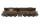Daily passes the same track section 8 pairs of trains with average 2400 passagers. How many people average travel in one train?
2. Unknown integerFind the smallest integer that: divided by 2, the remainder is 1 divided by 3 the remainder is 2, divided by 4 remainder is 3, ... divided by eight reminder is 7, by 9 reminder is 8.
3. ICE train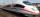German runways test a new ICE train between Munich and Berlin. The train runs to Berlin at a slow speed of 100 km/h. Back from Berlin goes faster. How quickly did the train have to go on a return trip so that the total average train speed for both journeys
4. Ascent and descent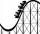Car goes 114 km track, which consists of ascent and descent at time of 1 hour 35 minutes. When climbing moves at the speed 48 km/h and downhill 25 m/s. What is the length of climb and descent?
5. Brick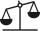Isosceles scale has on one side all brick and second weight 1 kg and 1/4 of brick. The balance is in equilibrium. What is the weight of a brick?
6. Brass tubeThe outer perimeter of brass tube (ρ = 8.5 g/cm3) is 38 cm. Its mass is 5 kg, length 54 cm. What is the pipe wall thickness?
7. Painting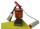Jožko with Fero painted the bench for four hours. How many hours would take work if four friends help him?
8. Automaker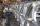The automaker now produces daily 4 new cars more than last year so the production of 360 cars will save just one full working day. How many working days to produce 360 vehicles needed last year?
9. Disinfecting solutionHow much distilled water is necessary to pour into 500 ml of 33% hydrogen peroxide solution to obtain 3% disinfecting solution?
10. Angles of the triangleABC is a triangle. The size of the angles alpha, beta are in a ratio 4: 7. The angle gamma is greater than the angle alpha by a quarter of a straight angle. Determine angles of the triangle ABC.
11. Class 8.AThree quarters of class 8.A went skiing. Of those who remained at home one third was ill and the remaining six were on math olympic. How many students have class 8.A?
12. Painters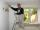The first team of painters would paint bridge in 15 days, the second in 10 days. After 3 days of working together second team goes out and continue only first team. How many days took second team to finish painting the bridge?
13. Angles ratioThe internal angles of a triangle are in ratio 1:4:5 What kind of triangle is it? (solve internal angles and write down and discuss)
14. Cows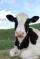20 cows has food for 60 days. We reduce the dose to two-thirds for 18 cows. How many days will be fed?
15. Sum-log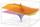The sum of two numbers is 32, the sum of their logarithms (base 10) is 2.2. Determine these numbers.
16. Self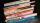A math text book is 2 2/9 inches thick. how many of these books will fit on a 120-inch self?
17. Brothers and sistersLenka has as many brothers as sisters. Her brothers have twice as many sisters as brothers. How many brothers and sisters are in the family?
18. Interest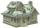What is the annual interest rate on your account if we put 32790 and after 176 days received 33939.2?
19. Czech crown salaryThe monthly salary of the employee is CZK 10,800. In the course of the year the bulk salary increased by CZK 500. Calculate which month (1 ... 12) was increased if its annual income was 133,600 CZK.
20. Soup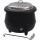On Monday we cook 25 pots and 10 boilers of soup. On Tuesday 15 pots and 13 boilers. On Wednesday 20 pots and on Thursday 30 boilers. On Monday and Tuesday was cooked the same amount of soup. How many times more soup cooked on Thursday than on Wednesday?

Do you have an interesting mathematical word problem that you can't solve it? Enter it, and we can try to solve it.

To this e-mail address, we will reply solution; solved examples are also published here. Please enter the e-mail correctly and check whether you don't have a full mailbox.

Please do not submit problems from current active competitions such as Mathematical Olympiad, correspondence seminars etc...